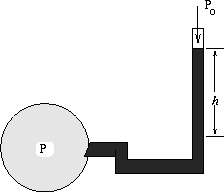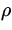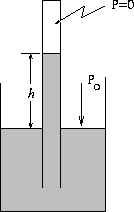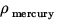# Pressure Measurements

Idea: It is possible to measure pressure by relating pressure differences to the variation in the height of a column of liquid. Two devices for measuring pressure are:

1. Open-tube manometerThe pressure P of the gas in the closed cylinder on the left in fig.9.5 is related to atmospheric pressure P0 (exerted on the top of the column of liquid on the right) by:

 P - P0 =gh (11)

whereis the density of the fluid in the closed tube. The pressure P of the gas is called the Absolute Pressure, to distinguish it from what is directly measured from the height of the column, namely the Gauge Pressure. Thus:

P absolute = P gauge + P0.

2. Mercury BarometerAtmospheric pressure is measured by ensuring that the pressure at the closed end of the barometer tube in Fig.9.6 is zero (i.e. it is vacuum). Thus

 P0 =gh (12)

whereis the density of the mercury.

It turns out that the pressure of 1 atmosphere (1 atm), is equivalent to a column of mercury exactly 0.76 m high at a temperature of 0 o C with g = 9.80665 m/s 2 and= 13.595 x 103 kg/m 3. From this information, we conclude that

 1  atm = 1.013 x 105  Pa = 101.3  kPa. (13)Next: Buoyant Forces Up: Solids and Fluids Previous: Variation of Pressure with